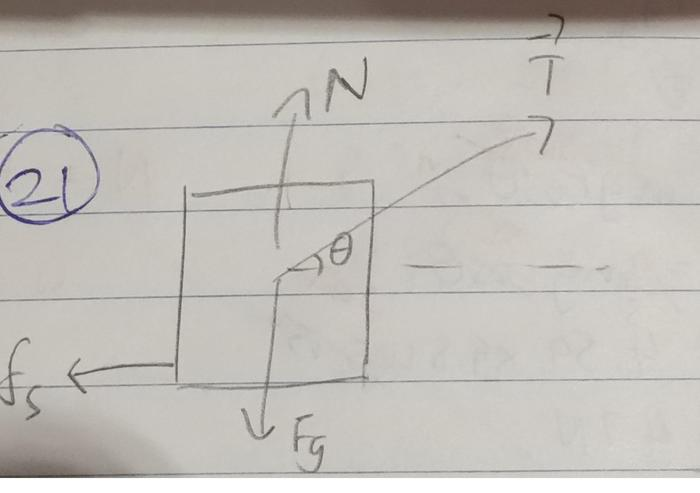# Friction problem

## Homework Statement

An initially stationary box of sand is to be pulled across a floor by means of a cable in which the tension should not exceed 1100 N. The coefficient of static friction between the box and the floor is 0.35. (a) What should be the angle between the cable and the horizontal in order to pull the greatest possible amount of sand, and (b) what is the weight of the sand and box in that situation?

## Homework Equations

f_s, max = mu_s*F_n

## The Attempt at a Solution

[/B]I would like some guidance on my thought process and maybe some hints on how to progress.

Defining up as +y and right as +x
Initially the box isn't moving so sum of F_x and sum of F_y is zero

From the FBD, for the y direction

F_n + T*sin(θ) - F_g = 0

so F_n = F_g - T*sin(θ), so subbing it into the static friction equation

so f_s, max = 0.35*(F_g - T*sin(θ))
= 0.35 (9.8m - 1100sin(θ)) N

From the FBD, for the x direction
T*cos(θ) - f_s = 0
T*cos(θ) = f_s
so f_s = 1100*cos(θ) N

Initially I thought θ = 0° would do, but then I thought maybe to "pull the greatest possible amount of sand", we want to find a θ value that best maximizes the cos part in the horizontal component of tension (so it overcomes the f_s, max) but at the same time best minimizes the sin part in f_s, max.

II have no idea how to do this besides maybe the derivative of f_s, max(θ) , but then I just end up with f's_max = cos(θ) = 0 and that is no help.

Maybe letting f_s = f_s,max could help, but I don't know how to deal with the pesky m or how I would solve for theta.

cnh1995
Homework Helper
Gold Member

## Homework Statement

An initially stationary box of sand is to be pulled across a floor by means of a cable in which the tension should not exceed 1100 N. The coefficient of static friction between the box and the floor is 0.35. (a) What should be the angle between the cable and the horizontal in order to pull the greatest possible amount of sand, and (b) what is the weight of the sand and box in that situation?

## Homework Equations

f_s, max = mu_s*F_n

## The Attempt at a Solution

[/B]I would like some guidance on my thought process and maybe some hints on how to progress.

Defining up as +y and right as +x
Initially the box isn't moving so sum of F_x and sum of F_y is zero

From the FBD, for the y direction

F_n + T*sin(θ) - F_g = 0

so F_n = F_g - T*sin(θ), so subbing it into the static friction equation

so f_s, max = 0.35*(F_g - T*sin(θ))
= 0.35 (9.8m - 1100sin(θ)) N

From the FBD, for the x direction
T*cos(θ) - f_s = 0
T*cos(θ) = f_s
so f_s = 1100*cos(θ) N

Initially I thought θ = 0° would do, but then I thought maybe to "pull the greatest possible amount of sand", we want to find a θ value that best maximizes the cos part in the horizontal component of tension (so it overcomes the f_s, max) but at the same time best minimizes the sin part in f_s, max.

II have no idea how to do this besides maybe the derivative of f_s, max(θ) , but then I just end up with f's_max = cos(θ) = 0 and that is no help.

Maybe letting f_s = f_s,max could help, but I don't know how to deal with the pesky m or how I would solve for theta.
Your FBD looks correct. Write an equation which connects the mass and angle theta. Use maximum friction and maximum normal reaction, since the block is on a horizontal surface.

Last edited:
haruspex
Homework Helper
Gold Member
find a θ value that best maximizes the cos part in the horizontal component of tension
No, you want the value that maximises m. Use the equations you have to find m as a function of theta (f_s eliminated).
maximum normal reaction,
How will maximising the normal reaction help? Wouldn't that be with a negative theta?

cnh1995
Homework Helper
Gold Member
How will maximising the normal reaction help? Wouldn't that be with a negative theta?
Sorry, I meant to say use 'total weight' of the box and sand. In absence of the pulling cable, normal reaction would be equal to the total weight. So I blindly used the term 'normal reaction' here. Normal reaction would be smaller when this cable is pulling the box..

ehild
Homework Helper

## Homework Equations

f_s, max = mu_s*F_n

## The Attempt at a Solution

so f_s, max = 0.35*(F_g - T*sin(θ))

From the FBD, for the x direction
T*cos(θ) = f_s

Maybe letting f_s = f_s,max could help
You derived the formula for the maximum static friction. The friction is horizontal, so it balances with the horizontal component of the tension. Make them equal 0.35*(F_g - T*sin(θ))=T*cos(θ), isolate F_g and find the angle where it is maximum.

Last edited: